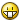## Recommended Posts

i created this function:

```Func IsPrimeNumber(\$num)
If IsNumber(\$num) = 0 Or \$num = 0 Then
Return -1
Else

Local \$pnum = 0
Local \$npnum = 0

\$i = 2
While \$i < Int(\$num)
\$ch = \$num / \$i
\$i = \$i + 1
If \$ch = Int(\$ch) Then
\$npnum = 1
Else
\$pnum = 1
EndIf
WEnd
If \$num = 2 Then
Return 1
ElseIf \$npnum = 1 And \$num <> 2 Then
Return 0
ElseIf \$pnum = 1 And \$npnum = 0 Then
Return 1
EndIf
EndIf
EndFunc```

it checks if a number is a prime number. if it is, the function returns 1, if not it returns 0. if you insert a string or 0 (which is not a real number) it returns -1

example:

```\$z = IsPrimeNumber(2)
If \$z = 1 Then
\$y = "TRUE"
ElseIf \$z = 0 Then
\$y = "FALSE"
ElseIf \$z = -1 Then
\$y = "NO NUMBER"
EndIf
MsgBox(0, "is prime?", \$y)```

this returns 1 and displays TRUE

but if i use it like this:

```\$log = @ScriptDir & "\Primzahlen.txt"

\$op = FileOpen(\$log, 1)
For \$i = 1 To 100
FileWriteLine(\$log, \$i)
EndIf
Next
FileClose(\$op)```

it doesnt write 2 in the log but as you know 2 returns 1 and is a prime number, too.

now my question: where´s the mistake?Mike

Edited by /\/\1|<3

##### Share on other sites

works now

correct function

Mike

Edited by /\/\1|<3

## Create an account

Register a new account

• ### Recently Browsing   0 members

×

• Wiki

• Back

• #### Beta

• Git
• FAQ
• Our Picks
×
• Create New...